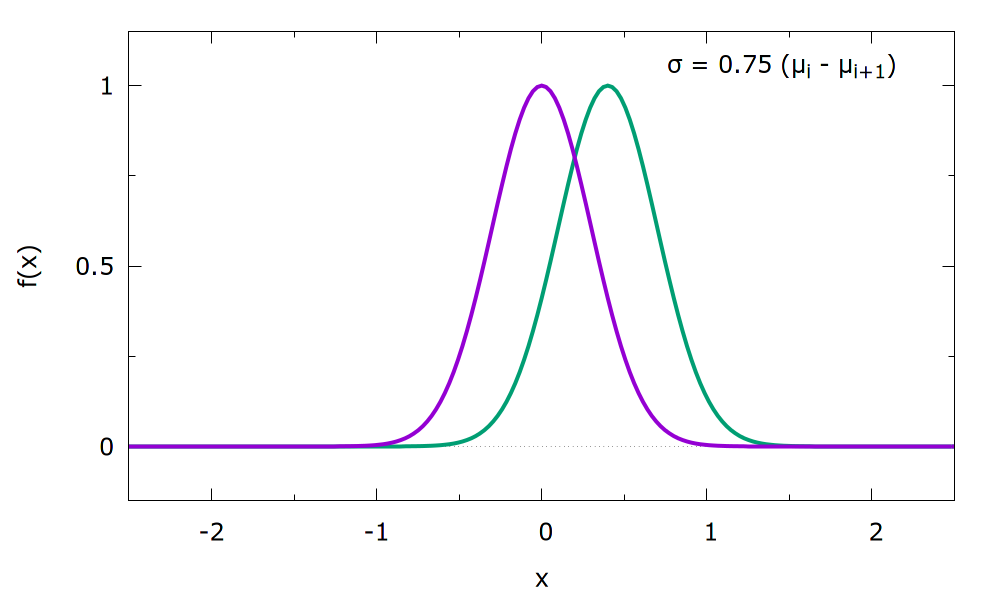BF_GAUSSIANS
 This is part of the ves module It is only available if you configure PLUMED with ./configure –enable-modules=ves . Furthermore, this feature is still being developed so take care when using it and report any problems on the mailing list.

Gaussian basis functions.

Attention
These basis functions do not form orthogonal bases. We recommend using wavelets (BF_WAVELETS) instead that do form orthogonal bases.

Basis functions given by Gaussian distributions with shifted centers defined on a bounded interval. See  for full details.

You need to provide the interval $$[a,b]$$ on which the bias is to be expanded. The ORDER keyword of the basis set $$N$$ determines the number of equally sized sub-intervalls to be used. On the borders of each of these sub-intervalls the mean $$\mu$$ of a Gaussian basis function is placed:

\begin{align} \mu_i = a + (i-1) \frac{b-a}{N} \end{align}

The total number of basis functions is $$N+4$$ as the constant $$f_{0}(x)=1$$, as well as two additional Gaussians at the Boundaries are also included.

The basis functions are given by

\begin{align} f_0(x) &= 1 \\ f_i(x) &= \exp\left(-\frac{{\left(x-\mu_i\right)}^2}{2\sigma^2}\right) \end{align}

When the Gaussians are used for a periodic CV (with the PERIODIC keyword), the sub-intervals are chosen in the same way, but only $$N+1$$ functions are required to fill it (the ones at the boundary coincide and the ones outside can be omitted).

It is possible to specify the width $$\sigma$$ (i.e. the standard deviation) of the Gaussians using the WIDTH keyword. By default it is set to the sub-intervall length. It was found that performance can be typically improved with a smaller value (around 75 % of the sub-interval length), although a too small overlap will prevent the basis set from working correctly at all.

The optimization procedure then adjusts the heigths of the individual Gaussians. To avoid 'blind' optimization of the basis functions outside the currently sampled area, it is often beneficial to use the OPTIMIZATION_THRESHOLD keyword of the VES_LINEAR_EXPANSION (set it to a small value, e.g. 1e-6)

As an example two adjacent basis functions (with the mentioned width choice of 75% of the sub-interval length) can be seen below. The full basis consists of shifted Gaussians in the full specified interval.Examples

The bias is expanded with Gaussian functions in the intervall from 0.0 to 10.0 using order 20. This results in 24 basis functions.

Click on the labels of the actions for more information on what each action computesbfG: BF_GAUSSIANS MINIMUMcompulsory keyword
The minimum of the interval on which the basis functions are defined. =0.0 MAXIMUMcompulsory keyword
The maximum of the interval on which the basis functions are defined. =10.0 ORDERcompulsory keyword
The order of the basis function expansion. =20


Because it was not specified, the width of the Gaussians is by default set to the sub-intervall length, i.e. $$\sigma=0.5$$. To e.g. enhance the overlap between neighbouring basis functions, it can be specified explicitely:

Click on the labels of the actions for more information on what each action computesbfG: BF_GAUSSIANS MINIMUMcompulsory keyword
The minimum of the interval on which the basis functions are defined. =0.0 MAXIMUMcompulsory keyword
The maximum of the interval on which the basis functions are defined. =10.0 ORDERcompulsory keyword
The order of the basis function expansion. =20 WIDTHThe width (i.e. =0.7

Glossary of keywords and components
Compulsory keywords
 ORDER The order of the basis function expansion. MINIMUM The minimum of the interval on which the basis functions are defined. MAXIMUM The maximum of the interval on which the basis functions are defined.
Options
 DEBUG_INFO ( default=off ) Print out more detailed information about the basis set. Useful for debugging. PERIODIC ( default=off ) Use periodic version of basis set. WIDTH The width (i.e. standart deviation) of the Gaussian functions. By default it is equal to the sub-intervall size.